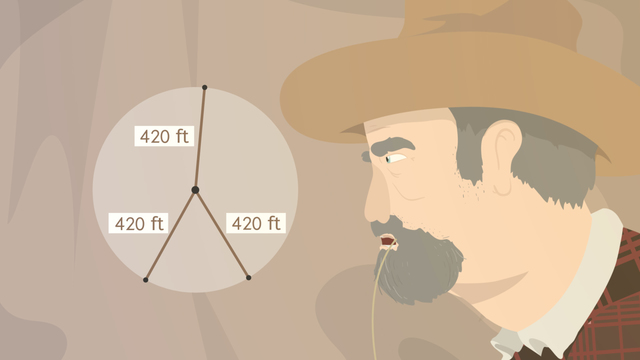# Understanding the Relationship between Circles and the number PiRating

Ø 5.0 / 1 ratings
The authorsTrentkay

## Basics on the topicUnderstanding the Relationship between Circles and the number Pi

After this lesson, you will be able to identify and define parts of a circle, plus explain the where the number pi comes from.

The lesson begins by teaching you the parts of a circle: radius, diameter, and circumference. It leads you to examine the ratio between a circle’s diameter and circumference, which is pi. It concludes with a definition of pi, which is often approximated by 22/7 or 3.14.

Learn about circles and the number pi by helping Farmer Johnson prove that aliens exist!

This video includes key concepts and vocabulary such as circle (a collection of points equidistant from the center); radius (a line from a circle’s center to edge), diameter (a line passing through a circle’s center, connecting two points on the edge), circumference (the distance around a circle), and pi (the ratio between a circle’s circumference and diameter).

Before watching this video, you should already be familiar with ratios and how to represent values as both fractions and decimals. You should also be comfortable with the concept of a circle.

After watching this video, you will be prepared to learn how to apply the number pi in other formulas, like the area of a circle.

Common Core Standard(s) in focus: 7.G.B.4, 7.G.B.6 A video intended for math students in the 7th grade Recommended for students who are 12-13 years old